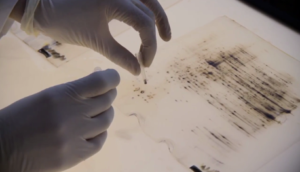Data analysis with correlation matrixData included in the ApreciseKUre Database are analyzed using classical statistical methods. The goal is to build up a dynamic model which describes how biological data are linearly correlated to each other. An algorithm has been developed to build up a refreshable correlation matrix based on Pearson’s correlation coefficient and p-value of each parameter and biomarker included in database (as you can see below). If Pearson’s coefficient ρ is greater than 0,3 in absolute values, the relationship between the two associated values are statistically significant. Additionally, the statistic reliability of Pearson’s index is evaluated by the p-value. A correlation matrix is a table containing the correlation coefficients between each variable and is used to examine the dependence between multiple variables at the same time. By visualizing every pair of variables, a complete statistical analysis can be obtained. This system gives a general picture of variable associations and its refresh feature allows the monitoring of reneweable relationships between the most recent data inserted in the database. In fact, Pearson’s index changes instantly as new datum is inserted through the appropriate database section; this change appears in the correlations matrix on the database homepage. For more information read the article: ApreciseKUre: an approach of Precision Medicine in a Rare Disease.

To gain access to the ApreciseKUre database click on “Database” below. For your first registration, send an e-mail to the staff.